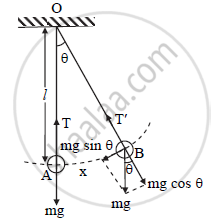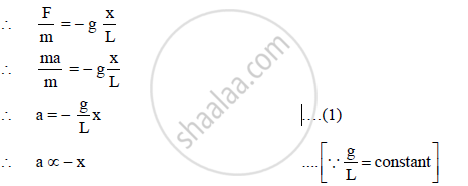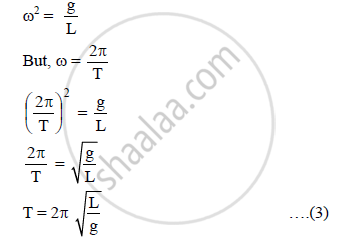HSC Science (Electronics) 12th Board ExamMaharashtra State Board
Share

# Show that Motion of Bob of the Pendulum with Small Amplitude is Linear S.H.M. Hence Obtain an Expression for Its Period. What Are the Factors on Which Its Period Depends? - HSC Science (Electronics) 12th Board Exam - Physics

ConceptSome Systems Executing Simple Harmonic Motion

#### Question

Show that motion of bob of the pendulum with small amplitude is linear S.H.M. Hence obtain an expression for its period. What are the factors on which its period depends?

#### Solution

To show the motion of the bob of the simple pendulum is S.H.M:1) Consider a simple pendulum of mass ‘m’ and length ‘L’.

L = l + r,

where, l = length of string

2) Let OA be the initial position of pendulum and OB, its instantaneous position when the string makes an angle Θ with the vertical.

In displaced position, two forces are acting on the bob:

a. Gravitational force (weight) ‘mg’ in the downward direction.

b. Tension T' in the string.

3) Weight ‘mg’ can be resolved into two rectangular components:

a. Radial component mg cos Θ along OB and

b. Tangential component mg sin Θ perpendicular to OB and directed towards mean position.

4) mg cos Θ is balanced by tension T' in the string, while mg sin Θ provides restoring force.

∴ F = - mg sin Θ

where, negative sign shows that force and angular displacement are oppositely directed. Hence, restoring force is proportional to sin Θ instead of Θ. So, the resulting motion is not S.H.M.

5) If Θ is very small then,

sin Θ ≈ Θ = x/L

∴ F = -"mg" x/LHence, the motion of the bob of a simple pendulum is simple harmonic.

Expression for the time period:

In S.H.M,

a = - ω2 x ….(2)

Comparing equations (1) and (2),Equation (3) represents the time period of the simple pendulum. Thus the period of a simple pendulum depends on the length of the pendulum and acceleration due to gravity.

Is there an error in this question or solution?

#### APPEARS IN

2015-2016 (July) (with solutions)
Question 4.3 | 4.00 marks

#### Video TutorialsVIEW ALL 

Solution Show that Motion of Bob of the Pendulum with Small Amplitude is Linear S.H.M. Hence Obtain an Expression for Its Period. What Are the Factors on Which Its Period Depends? Concept: Some Systems Executing Simple Harmonic Motion.
S